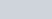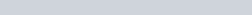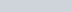ChemTalk

# Normality and Other Units of Concentration## Core Concepts

There are many ways to measure concentration besides molarity. In this article we will discuss normality, specifically how it is different from molarity, as well as its application and equations used to find the normality of a substance. The overarching concept is the different aspects of concentrations in solutions and how these are expressed through units like percent, PPM, PPB, molarity, and normality.

## Concentration Vocabulary

When discussing normality in chemistry it is important to know some keywords to understand the broader context of this concept.

• Equivalent: The amount of a substance that can react with or replace a certain amount of another substance in a chemical reaction.
• Equivalent weight: The weight of a substance that is equivalent to one mole of protons, electrons, or other reactive species in a chemical reaction.
• Hydrogen Ion (H+) and Hydroxide Ion (OH-): Ions that play a key role in acid-base reactions and are often used to calculate normality
• Strong Acid and Strong Base: Substances that completely dissociate into ions when dissolved in water, leading to higher normality values due to the higher number of ions produced.
• Titration: A technique used to determine the concentration of a substance in a solution by reacting it with a solution of known concentration (titrant) until the reaction is complete.

## Concentration: Normality

Normality (N) is a way of expressing the concentration of a solute as one gram or mole equivalent present in one liter of solution. Normality is not to be confused with molarity, which refers to the measure of concentration of a solute in terms of moles per liter of a solution. There is a relationship between the two, however, it depends on the type of reaction and number of moles of ions being either produced or consumed in a reaction. Normality is useful for reactions that include acids, bases, redox reactions, and titration. While molarity focuses on the quantity of moles present in a solute, normality considers both the quantity and the reactivity.## Normality and Reaction Units

Since normality depends on reaction equivalents, a solution of NaCl can only have one molarity. But there can be multiple normality values depending on the reaction in which it participates. The first step to finding a substance’s normality is to find its reaction units, n. To find the reaction units of all the reagents in a reaction, you follow these 2 steps:

1. Find the highest stoichiometric coefficient among the reactants.
2. Set the reaction units of the reactant with this coefficient as n=1.
3. Divide this same coefficient by each of the other coefficients to get those reactant’s n.

For example, take this hypothetical reaction:First, we find that D has the highest stoichiometric coefficient of 12. Thus, we find that D has 1 reaction unit.Finally, to get the remaining reaction units, we divide 12 by the coefficients of A, B, and C to get the reaction units of those reagents:

(1)From there, we simply need to divide each value of n by the liters in the solution of the reaction to find each reactant’s normality.

## Concentration: PPM and PPB

PPM stands for parts per a million. PPB stands for parts per billion. These are different concentration units that are usually used for very dilute substances. When describing a so centrations in ppm, it can be thought of literally as one part per a million.

1 mg/kg = 1 ppm. When you do the conversion of 1 kg to mg, you get 1 kg = 1,000,000 mg. If you have 1 mg of a substance within 1,000,000 mg (or 1 kg) or another substance, than then there is 1 mg per millions milligrams (1ppm)

Parts per billion is the same idea as parts per million except it is out of a billion. So 1mg per 1000 kg would be 1 ppb.

These units are often used in environmental measurements. Such as the concentration of a pollutant in water, or a contaminant in the air. One of the key pieces of evidence of climate change is the concentration of carbon dioxide in the air. The concentration of CO2 is report in ppm typically.

## Concentration: w/w and v/v Percent

W/w percent is a measurement of concentration that measure the percentage of the analyte of interest in the whole. It may be written several different ways, including %w/w, w/w% or weight percent. For example, if you have 30 g of acid in 70 g of water, then the solution is acid 30% w/w. This comes from (30g / (30 g + 70g)) *100.

V/V percent is very similar as %w/w except uses volume instead of weight. It can also be abbreviated in different forms such as %v/v, v/v% and volume percent.